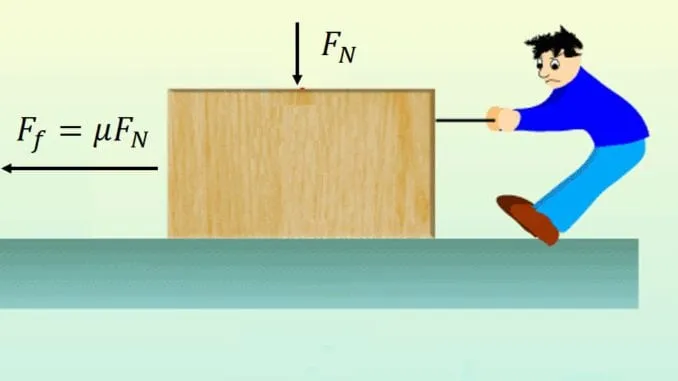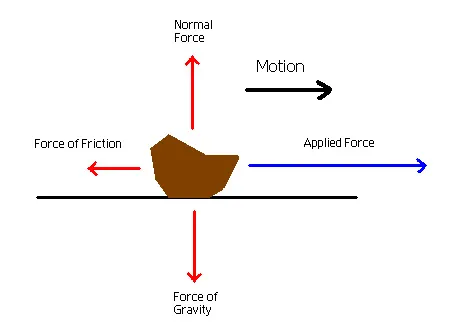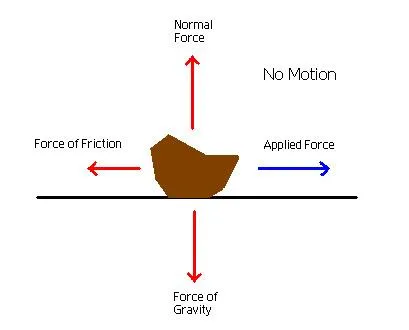# Physics - Classical mechanics - Contact forces and frictionHello it's a me again drifter1! Today we continue with the Classical mechanics branch of Physics by getting into contact forces and friction. I already talked a little bit about friction in my post about Newton's 3rd law. Knowing the 3rd law will be pretty important for contact forces and so I suggest you to check it out if you haven't already! So, without further do, let's get started!

## Small recap

In my previous posts we covered Newton's laws.Sir Isaac Newton

The first and second law cover the motion of an object.

When the total force applied it zero then the object keeps it's current state and either stays at rest or moves with a constant velocity, cause we have zero acceleration. (1st law)

When the total force is not zero then the object has a acceleration and we use the equation:

F = m a    (2nd law)

Note that an object might have a different kind of motion for each of the component vectors for the axes and so we might use both of these laws on the same object!

Lastly, the 3rd law tells us that for each action there is always a reaction. This means that when we apply a force to an object this object applies the same (opposite) force to us. The sum of those two forces is zero and not the total force applied to us or the object! (but it can be if other forces also are in the game)

So, let's now get into contact forces.

## Contact Forces

When two objects interact directly through contact, then the interaction forces are contact forces.

The two most important contact forces are:

• vertical forces (that the ground applies to us when we stand on it for example)
• friction (that causes slip and slide that slow us down)

Let's get into each of these more specifically.

## Vertical force (N)

When an object is at rest or slides, and so is on top of a surface it applies a force equal to it's weight (exactly the weight if it a horizontal plane, the vertical component to the surface when on a slope) to that surface. Because of the 3rd law we know that the surface also applies the same force back to the object.

We call this force N and this force is parellel to the "slip" surface.

From that you can clearly see that the vertical force and friction will always be perpendicular to each other (will have a 90 degree angle to each other).

So, N is not so difficult to be calculated, cause we just have to find the right vector component of the object's weight and set it equal to N, because the object stays at rest on that vertical axis and so Newton's 1st law applies.

## Friction

Well, friction has a connection to the vertical force N.

I already told you last time that the friction is equal to:

T = μ*N

where T is the friction, μ is the surface "slip" factor and N is the vertical force.

From that we can see that we will need more force when the vertical force is larger and so the object "weight's" more. We will also need less force when the surface is more "slippy", which means that μ is close to zero.

There are two types of friction:

• kinetic friction (Tk) that is applied when the object is moving
• static friction (Ts) that is applied when the object is at restThe kinetic friction is equal to:

Tk = μk * N

where μk is the factor of kinetic friction.

Kinetic friction is always the same when the object is moving!

Of course the object doesn't slope on it's whole surface, and the surface will also not be exactly the same, but we suppose that this doesn't need to be calculated! We just estimate by using the "perfect" scenario!The static friction is:

Ts <= μs * N

where μs it the factor of static friction.

Static friction shows us how much force we have to apply until the object starts moving on a surface. This means that the static friction might also be zero, when no force is applied.

μs * N is the maximum value of static friction that we can have.

At that exact moment where we surpass it we start getting kinetic friction.

So, while the object doesn't move, when applying force to it the 1st law is true and so ΣF = 0.

There we can calculate the static friction factor.

The static and kinetic friction factors are not equal (but they can be).

The first one is mostly bigger and so we have to apply a little more force to make an object start moving then keeping it in motion!

An interesting diagram is this:You can see that the applied force from the object to us (friction) is first growing and after the object starts moving we see a decrease in that force and the friction stays then almost the same!

Something worth mentioning is that we also have other type of friction, like the friction that a rotating wheel has The friction Tr with a factor μr is much smaller then the other frictions and that's why we use wheel's for vehicles etc. to have the least amount of friction possible!

### Image Sources:And this is actually it for today! I hope that you enjoyed it!

Next time we will get into the Dynamics of Circular Motion!

Bye!

H2
H3
H4
3 columns
2 columns
1 column